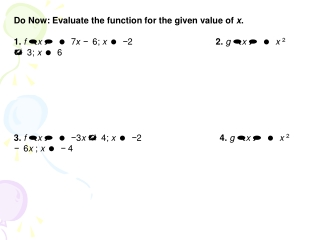DownloadDownload PresentationAlgebra II

# Algebra II

Download Presentation## Algebra II

- - - - - - - - - - - - - - - - - - - - - - - - - - - E N D - - - - - - - - - - - - - - - - - - - - - - - - - - -
##### Presentation Transcript

1. Algebra II 4.1: Graphing Polynomial Functions Objective: To identify polynomials, and to use several techniques to get rough sketches.

2. Vocabulary • What is a monomial? • An expression that is either a number, a variable, or the product of a number and one or more variables

3. Vocabulary • What is a Polynomial? • A monomial or a sum of monomials

4. A polynomial function is in standard form when its terms are written in descending order of exponents from left to right.

5. Vocabulary • Polynomial Function = a function in the form: n is the degree or the highest exponent. is the leading coefficient. is the constant term.

6. the exponents are all whole numbers • What is a whole number? • the coefficients are all real numbers • What is a real number?

7. Common Polynomial Functions